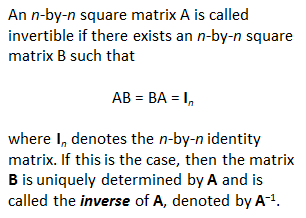# Matrix InversionThis program performs the matrix inversion of a square matrix step-by-step. The inversion is performed by a modified Gauss-Jordan elimination method. We start with an arbitrary square matrix and a same-size identity matrix (all the elements along its diagonal are 1). We perform operations on the rows of the input matrix in order to transform it and obtain an identity matrix, and

perform exactly the same operations on the accompanying identity matrix in order to obtain the inverse one.

If we find a row full of zeros during this process, then we can conclude that the matrix is singular, and so cannot be inverted.

We're exposing a very naive method, just as was performed in the old-Basic- style. Naturally, Matlab has appropriate and fast instructions to perform matrix inversions (as with 'inv' or '\', for example), but we want to explain the Gauss-Jordan concept and show how nested loops and control flow work.

First, we develop a function like this (let's assume we save it as 'mat_inv2.m'):

function b = mat_inv2(a)
% Find dimensions of input matrix
[r,c] = size(a);

% If input matrix is not square, stop function
if r ~= c
b = [];
return
end

% Target identity matrix to be transformed into the output
% inverse matrix

b = eye(r);

%The following code actually performs the matrix inversion by working
% on each element of the input

for j = 1 : r
for i = j : r
if a(i,j) ~= 0
for k = 1 : r
s = a(j,k); a(j,k) = a(i,k); a(i,k) = s;
s = b(j,k); b(j,k) = b(i,k); b(i,k) = s;
end
t = 1/a(j,j);
for k = 1 : r
a(j,k) = t * a(j,k);
b(j,k) = t * b(j,k);
end
for L = 1 : r
if L ~= j
t = -a(L,j);
for k = 1 : r
a(L,k) = a(L,k) + t * a(j,k);
b(L,k) = b(L,k) + t * b(j,k);
end
end
end
end
break
end
% Display warning if a row full of zeros is found
if a(i,j) == 0
disp('Warning: Singular Matrix')
b = 'error';
return
end
end
% Show the evolution of the input matrix, so that we can
% confirm that it became an identity matrix.
a

And then, we can call it or test it from any other script or from the command window, like this:

% Input matrix
a = [3  5  -1   -4
1  4  -.7  -3
0 -2   0    1
-2  6   0   .3];

% Call the function to find its inverse
b = mat_inv2(a)

% Compare with a result generated by Matlab
c = inv(a)

Matlab produces this response:

First, we see how the original matrix was transformed into an identity matrix:

a =
1     0     0     0
0     1     0     0
0     0     1     0
0     0     0     1

Then, our function shows its result:

b =
0.6544   -0.9348   -0.1912    0.0142
0.1983   -0.2833   -0.1034    0.1558
0.3683   -1.9547   -4.2635   -0.4249
0.3966   -0.5666    0.7932    0.3116

Finally, this is the inversion produced by an instruction from Matlab (inv(a)):

c =
0.6544   -0.9348   -0.1912    0.0142
0.1983   -0.2833   -0.1034    0.1558
0.3683   -1.9547   -4.2635   -0.4249
0.3966   -0.5666    0.7932    0.3116

Another example:

% Input matrix
a = [1 1
1 1];

% Call the function to find its inverse
b = mat_inv2(a)

% Compare with a result generated by Matlab
c = inv(a)

And Matlab display is:

Warning: Singular Matrix
b =
error

Warning: Matrix is singular to working precision.
> In test_mat_inv at 42

c =
Inf   Inf
Inf   Inf

In this case, our algorithm found a singular matrix, so an inverse cannot be calculated. This agrees with what Matlab found with its own built-in function.

If you are interested in a Modified Gauss-Jordan Algorithm, you can see this article.

From 'Matrix Inversion' to home

From 'Matrix Inversion' to 'Linear Algebra'

Examples on Flow Control

 Top Flow Control